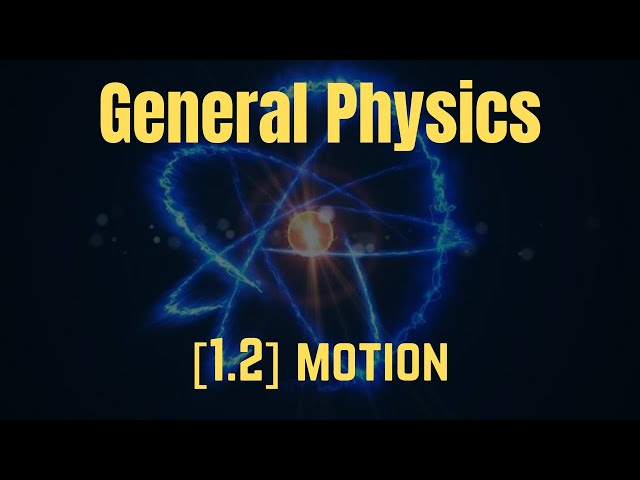# Physics [Syllabus 1.2] Motion: Understanding Speed, Velocity, and AccelerationTLDR Speed is the distance covered per second, while velocity takes into account both distance and direction, and objects experience constant acceleration due to gravity until reaching terminal velocity.

## Timestamped Summary

• 🏃
00:00
Speed is the distance covered per second, while velocity is the displacement per second, taking into account both distance and direction, and the overall displacement and velocity of a round trip is zero.
• 📚
04:40
Acceleration is the change in velocity over time, with positive indicating an increase and negative indicating a decrease, while speed is distance over time and velocity is displacement over time.
• 🌍
07:01
Objects falling experience constant acceleration due to gravity, but air resistance eventually causes them to reach a constant velocity known as terminal velocity.
• 📊
10:44
The displacement time graph can be used to calculate velocity by looking at the gradient, with a straight line indicating no movement, a downward slope indicating return to starting point, and a wavy line indicating changing speed.
• 📊
14:19
The speed-time graph can be used to calculate distance and acceleration, making it important for exam calculations.
• 🚗
16:26
The car accelerates to its maximum speed and maintains a constant speed with no acceleration, while a runner accelerates, reaches maximum speed, maintains it, and then decelerates to a stop.
• 🪂
18:51
The skydiver experiences a varying acceleration due to gravity, eventually reaching a constant speed when the forces of gravity and air resistance are balanced.
• 👍
21:39
The speaker offers help and resources for those interested in physics, biology, and chemistry on their Patreon channel, and will be covering past papers in future videos.

## Q&A

• What is the difference between speed and velocity?

Speed is the change in distance per second, while velocity takes into account both distance and direction of travel.

• What is displacement?

Displacement is the total distance and direction of travel.

• What is terminal velocity?

Terminal velocity is the constant velocity reached by an object when the gravitational force and air resistance are equal.

• How is acceleration calculated?

Acceleration is calculated as the change in velocity per second.

• How can speed-time graphs be used?

Speed-time graphs can be used to calculate distance traveled and determine acceleration.Report the articleThank you for the feedback